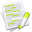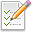#wx.Image.RGBValue¶

A simple class which stores red, green and blue values as 8 bit integers in the range of 0-255.

##Class Hierarchy¶Inheritance diagram for class RGBValue:

##Methods Summary¶

 __init__ Constructor for wx.Image.RGBValue, an object that contains values for red, green and blue which represent the value of a color.

##Properties Summary¶

 blue A public C++ attribute of type int. green A public C++ attribute of type int. red A public C++ attribute of type int.

##Class API¶

class wx.Image.RGBValue(object)

Possible constructors:

RGBValue(r=0, g=0, b=0)

A simple class which stores red, green and blue values as 8 bit

integers in the range of 0-255.

### Methods¶

__init__(self, r=0, g=0, b=0)

Constructor for wx.Image.RGBValue, an object that contains values for red, green and blue which represent the value of a color.

It is used by wx.Image.HSVtoRGB and wx.Image.RGBtoHSV , which convert between HSV color space and RGB color space.

Parameters
• r (int) –

• g (int) –

• b (int) –

### Properties¶

blue

A public C++ attribute of type int.

green

A public C++ attribute of type int.

red

A public C++ attribute of type int.